# #夏日挑战赛# 3.7 Counter组件 原创精华

1收藏

[ 本文正在参加星光计划3.0–夏日挑战赛 ] 本节介绍一个比较简单的计数器组件Counter，可提供相应的增加或者减少的计数操作。

#### 1.接口

Counter组件的接口不需要任何参数。

``````Counter()
``````

#### 2.事件

``````onInc(callback: () => void) 监听数值增加事件。
onDec(callback: () => void) 监听数值减少事件。
``````

#### 示范代码

``````  @State value: number = 0 // 数值
private step: number = 10 // 步长
``````

``````Row(){
TextBody1({text: \$r("app.string.title_quantity")}) // 标题
Blank()
Counter() {
Text(this.value.toString()).fontSize('20fp')
}
.backgroundColor(\$r("app.color.success"))  // 计数器组件无法设置背景色
.onInc(() => { // 监听数值增加事件
this.value += this.step
})
.onDec(() => { // 监听数值减少事件
this.value -= this.step
})
}.width('100%')
``````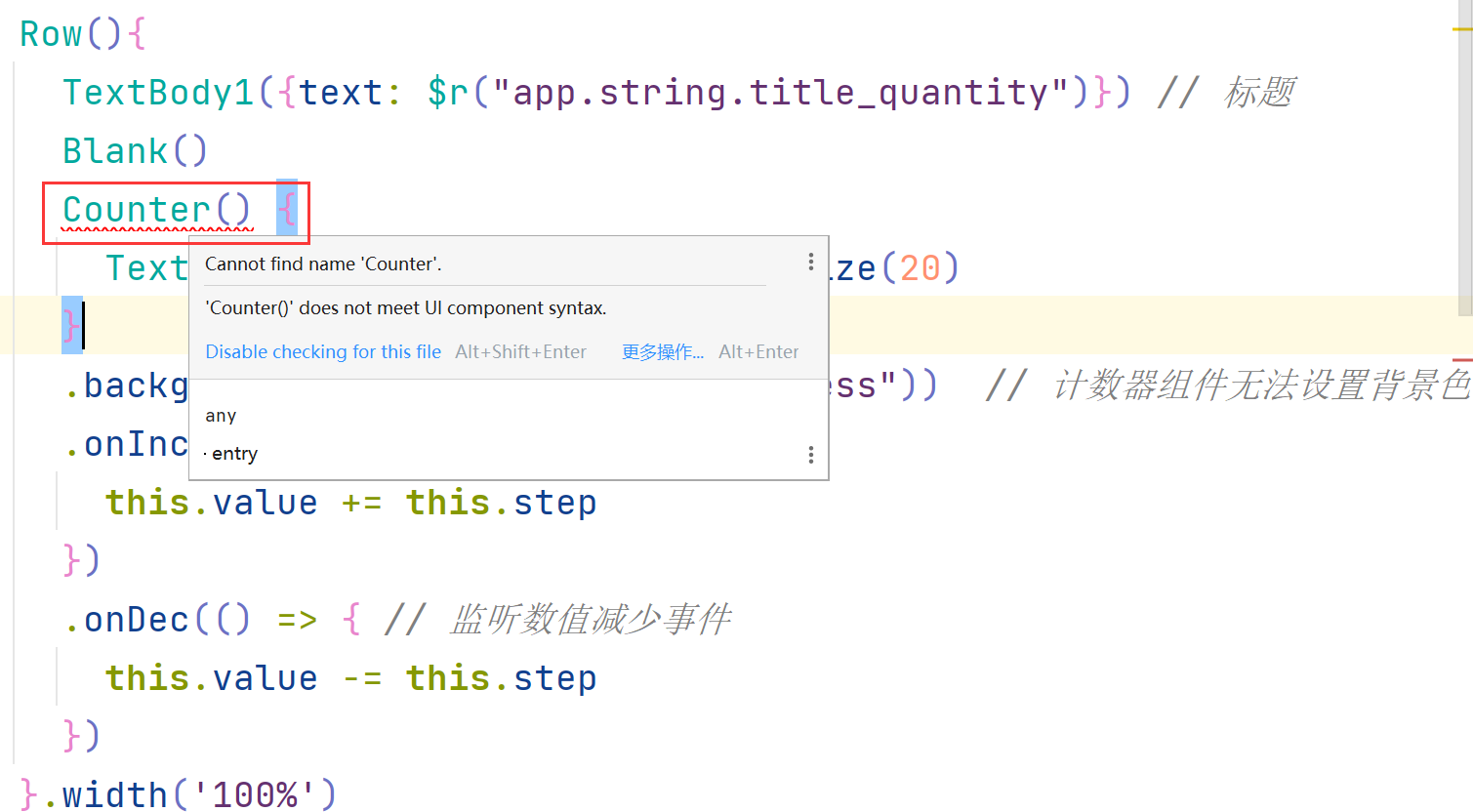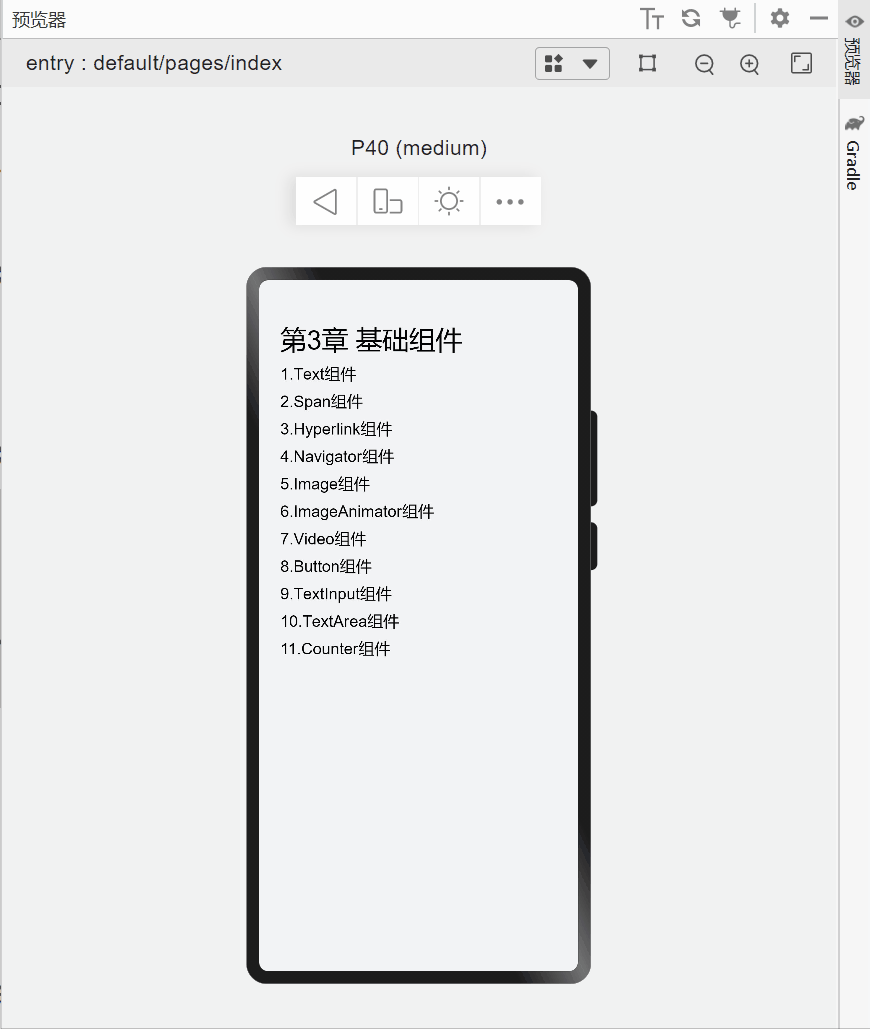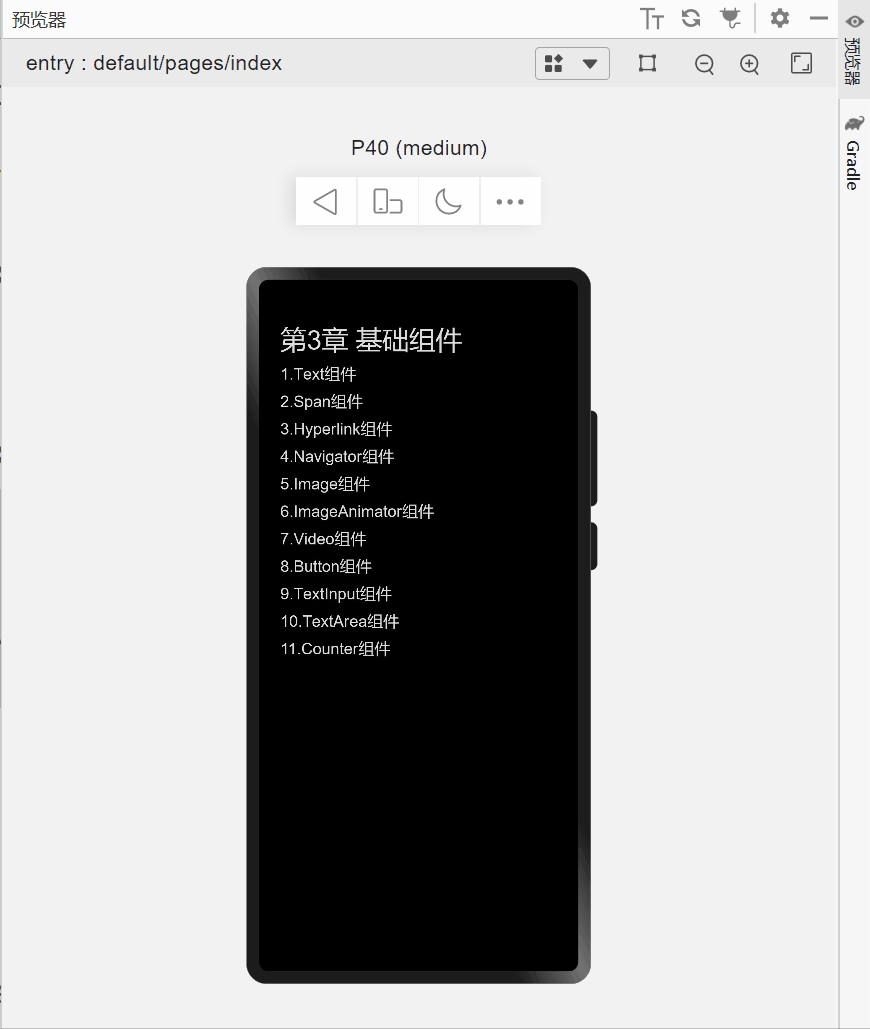#### 注意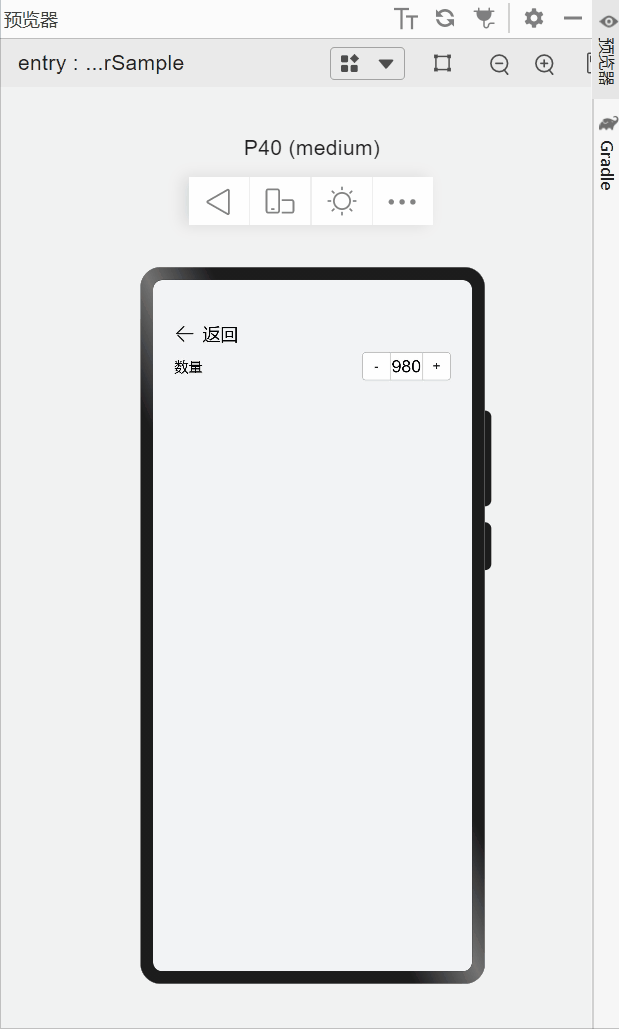``````   .width(150) // 设置一个宽度
``````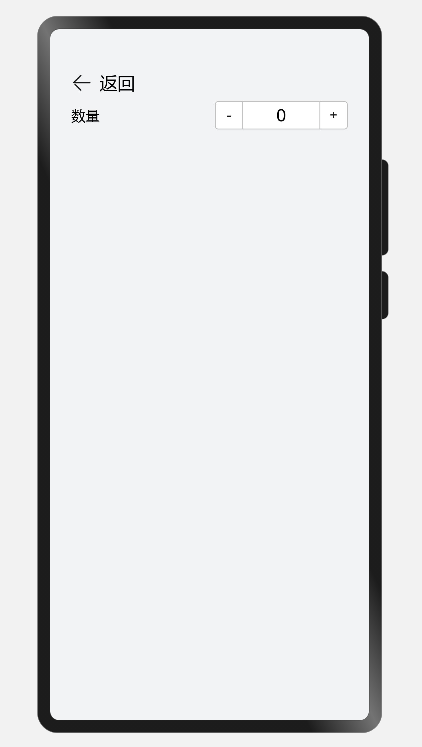``````import {H8, TextBody1} from './index'

/**
* 3.7 Counter组件
* 计数器组件，提供相应的增加或者减少的计数操作。
* 可以包含子组件。
*
* 事件:
*  不支持通用事件和手势， 仅支持如下事件：
*  onInc(callback: () => void) 监听数值增加事件。
*  onDec(callback: () => void) 监听数值减少事件。
*/
@Entry
@Component
struct CountSample {
@State value: number = 0 // 数值
private step: number = 10 // 步长
build() {
Column({space:8}) {
// 返回首页
Row({space:8}){
Image(\$r("app.media.ic_back")).width(24).height(24)

Navigator({ target: 'pages/index', type: NavigationType.Back }) {
H8({text:'返回'})
}
}

Row(){
TextBody1({text: \$r("app.string.title_quantity")}) // 标题
Blank()
Counter() {
Text(this.value.toString()).fontSize('20fp')
}
.width(150) // 设置一个宽度
.backgroundColor(\$r("app.color.success"))  // 计数器组件无法设置背景色
.onInc(() => { // 监听数值增加事件
this.value += this.step
})
.onDec(() => { // 监听数值减少事件
this.value -= this.step
})
}.width('100%')
}
.width('100%')
.height('100%')
.padding({top: \$r("app.float.spaceTop"), bottom:\$r("app.float.spaceBottom"), left:\$r("app.float.spaceLeft"), right: \$r("app.float.spaceRight")})
.backgroundColor(\$r("app.color.appBg"))
.alignItems(HorizontalAlign.Start)
}
}
``````